• 使用指向结构体数组的指针变量与指向一维数组的指针变量类似，可以修改指针变量的值（const可以限定） 代码示例： #include <stdio.h> typedef struct _test { int i; double d; char str; }Test,*...
使用指向结构体数组的指针变量与指向一维数组的指针变量类似，可以修改指针变量的值（const可以限定）
代码示例：
#include <stdio.h>
typedef struct _test
{
int i;
double d;
char str;
}Test,*pTest;

int main()
{
int i;
Test te={{1,1.1,"111"},{2,2.2,"222"},{3,3.3,"333"},{4,4.4,"444"}};

pTest p,q;
p=te;
for(i=0;i<2;i++)
{
printf("%d,%.2f,%s\n",(p+i)->i,(p+i)->d,(p+i)->str);
}
for(q=te+2;q<te+4;q++)
{
printf("%d,%.2f,%s\n",q->i,q->d,q->str);
}
return 0;
}

代码中p+i表达式的值实际是下标i的结构体元素的地址。


展开全文c语言
• C语言结构体数组+结构体类型指针+指向结构体数组的指针+typedef类型结构体数组两种结构体数组的定义形式用sizeof运算符求结构体数组所占字节数结构体类型指针指向结构体数组的指针typedef类型 结构体数组 如果要处理...
上一篇文章：C语言结构体类型定义+结构体变量的定义与使用及其初始化+结构体变量作为函数参数
C语言结构体数组+结构体类型指针+指向结构体数组的指针+typedef类型结构体数组两种结构体数组的定义形式用sizeof运算符求结构体数组所占字节数结构体数组初始化结构体数组作为函数的参数查找符合条件的人数(一)结构体类型指针 和 指向结构体数组的指针指向结构体变量的指针的定义利用结构体指针引用结构体变量成员指向结构体数组的指针查找符合条件的人数(二)typedef类型学生成绩排序修改学生成绩下一篇文章
结构体数组
如果要处理多个类型相同的结构体数据，就需要定义结构体数组
两种结构体数组的定义形式
结构体数组定义的方法和结构体变量定义的方法一样，只不过加了一个数组的长度而已
第一种：和结构体类型同时定义，例如：
struct student
{
int sno;
char sname;
int sage;
char depmt;
float cscore;
}s1;//定义了一个结构体数组，数组名为s1，数组的长度为10

第二种：和结构体类型分开定义，如：
struct student
{
int sno;
char sname;
int sage;
char depmt;
float cscore;
};//不要忘记最后的分号
main()
{
struct student s1;//在主函数中定义结构体数组，数组名为s1，数组长度为10
...
}

用sizeof运算符求结构体数组所占字节数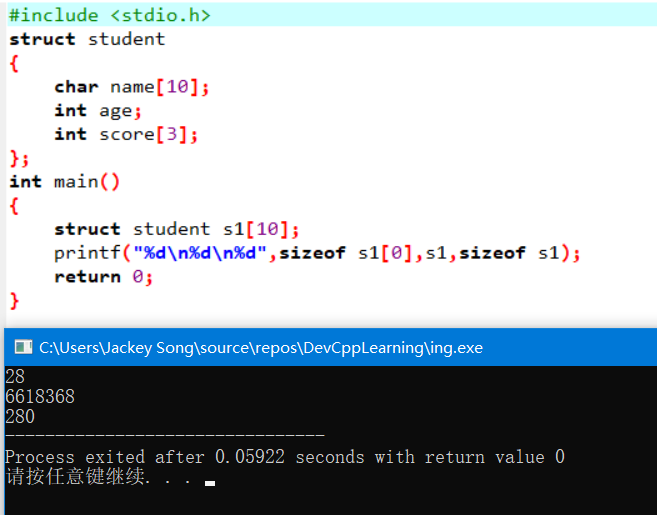char name 占用10个字节，int age 占用4个字节，int score占用3*4=12个字节，加起来总共是26个字节，可是上面输出的是28个字节，多出两个字节。这是因为在编译系统中，结构体类型存在字节边界的要求。而不同编译器字节边界的要求又是不一样的，上面这个案例我使用的是DevC++编译器，然而试验了一番之后，我并没能发现DevC++编译器字节边界要求的规律，反而把我给整懵圈了，如下：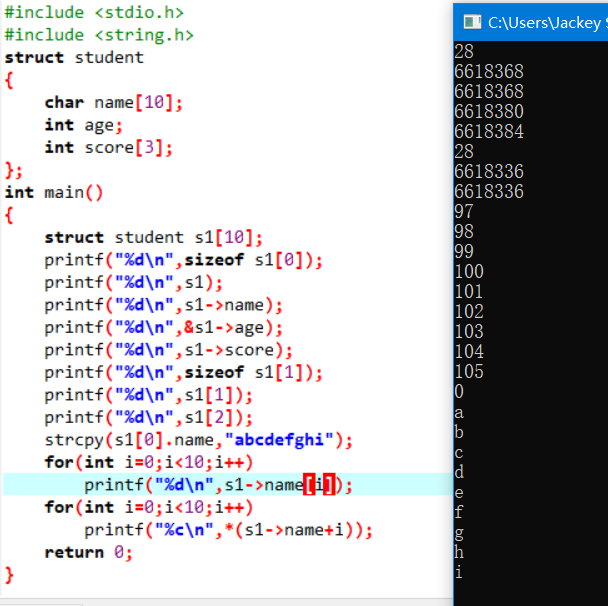虽然它的内存地址分配规律我没能搞懂，但是在使用的时候，依然可以正常用指针来操纵内存地址，进而操纵对应内存地址中的数据。 如下：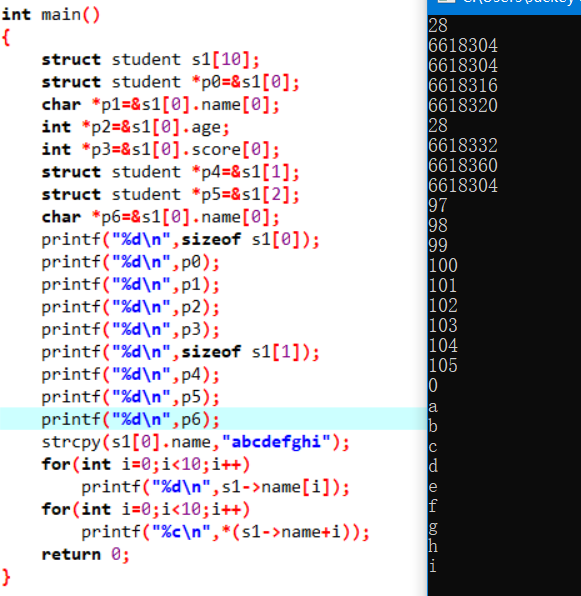结构体中的内存地址分配规律，相对于普通的数据类型的内存地址分配规律，要更加复杂一些，而且不同的编译器也不一样。
在VC编译器中，各成员变量在内存中的起始地址相对于结构体变量(或数组)的起始地址的偏移量，必须为该变量的类型所占字节数的倍数。如果某成员的偏移量不是该类型的整数倍，则VC编译系统会自动填充一定的字节数，以保证字节边界的要求。同时VC为了确保结构体的大小为结构体中所占内存空间最大的成员类型的字节数的倍数，在为最后一个成员变量申请空间后，还会根据需要自动填充空缺的字节。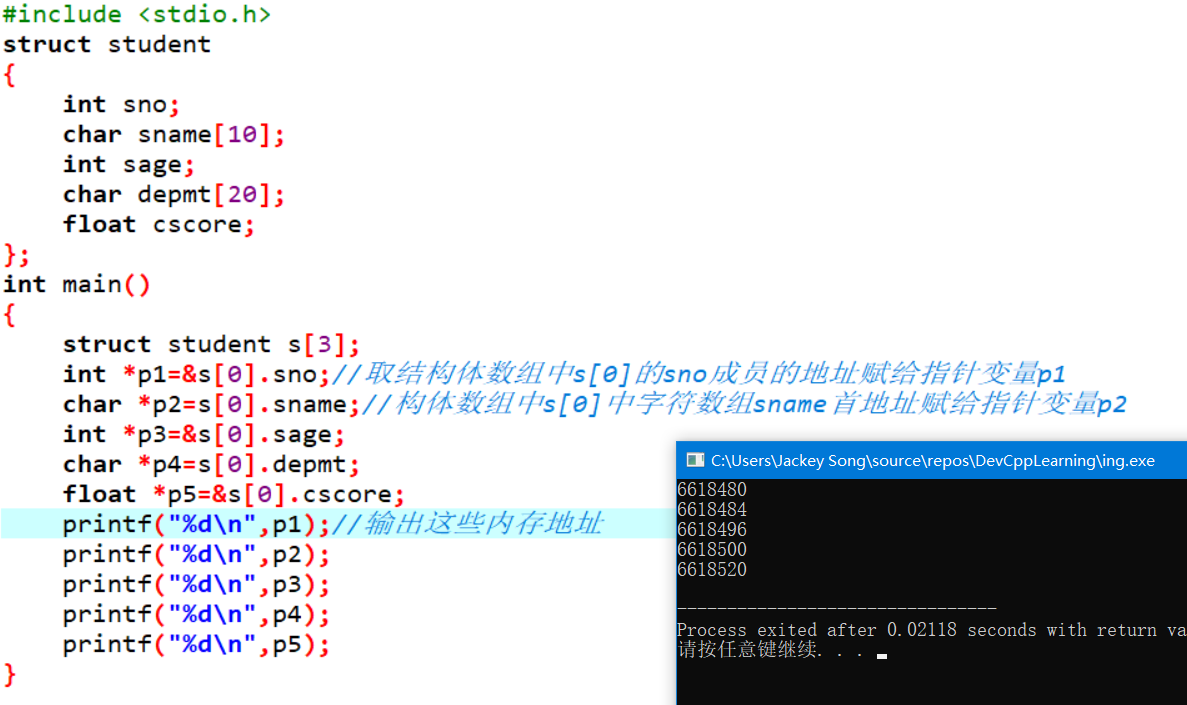内存地址相减得到各成员变量所占的字节数
注意：
访问结构体中的数据时，格式为结构体变量.结构体成员，这个时候使用的是点. 如果是一个指针变量p，p指向一个结构体类型，用指针来访问的话那么格式为指针变量->结构体成员，这个时候使用的是减号和大于号->，表示一个箭头，注意区分一下，使用的时候不要出错
结构体数组初始化
格式为：结构数组[n] = {{初值表1},{初值表2}, ... ,{初值表n}};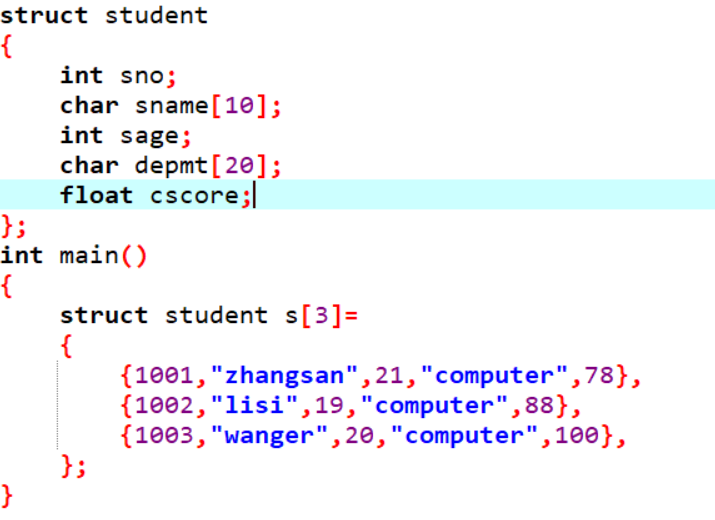用for循环输出结构体数组中的內容：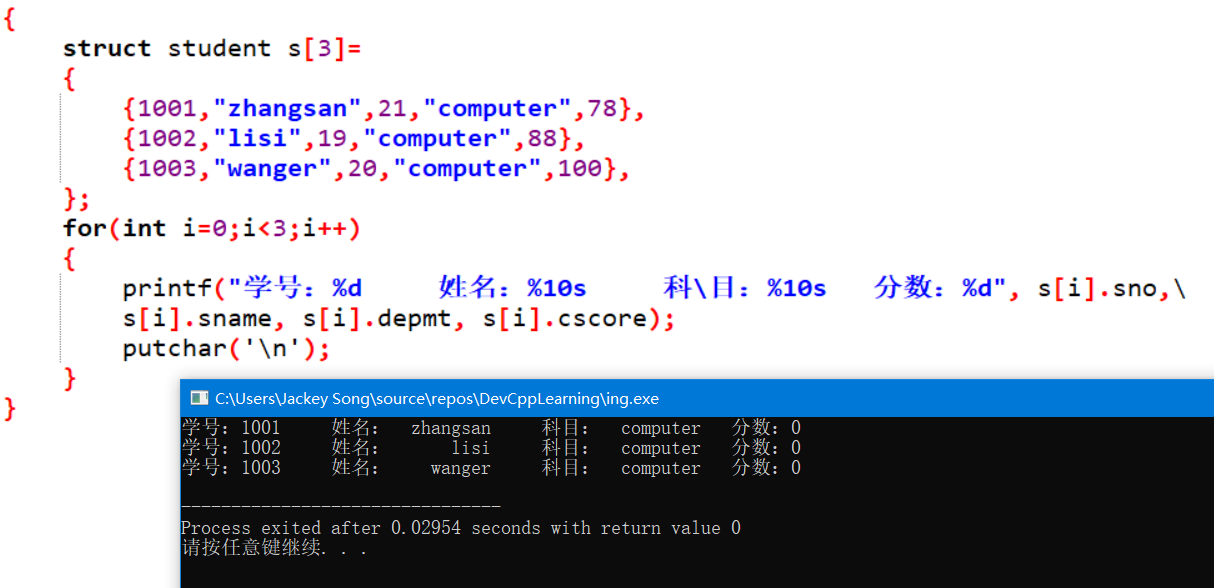当一个代码语句太长时，用反斜杠来进行代码换行
图中的代码有个字母打错了，导致最后的成绩输出全部是0，分数是float型的，而输出的时候却用了%d，应该用%f
结构体数组作为函数的参数
结构体数组作为函数的参数时，既可以作为形参，也可以作为实参，和普通数组一样。实参到形参的传递为地址传递。
查找符合条件的人数(一)
#include <stdio.h>
#define N 10
struct student
{
int no;
char name;
int score;
};
int fun(struct student s[],struct student b[],int m,int n);
void output(struct student x[],int n);
int main()
{
struct student s[N]=
{
{1001,"zhangsan",79},
{1002,"lisi",87},
{1003,"wanger",83},
{1004,"mazi",86},
{1005,"wangwu",89},
{1006,"wangliu",75},
{1007,"liwu",84},
{1008,"zhangwu",93},
{1009,"zhangqi",85},
{1010,"masan",97},
};
int m,n,k;
struct student a[N];
printf("please input range in m,n:\n");
scanf("%d%d",&m,&n);
printf("The original data:\n");
output(s,N);
k=fun(s,a,m,n);
printf("The student that score in %d & %d is:\n",m,n);
output(a,k);
return 0;
}
int fun(struct student s[],struct student b[],int m,int n)
{
int i,k=0;
for(i=0;i<N;i++)
{
if(s[i].score>=m&&s[i].score<=n)
b[k++]=s[i];
}
return k;
}
void output(struct student x[],int n)
{
int i;
printf(" no          name       score\n");
for(i=0;i<n;i++)
printf("%d   %10s       %d\n",x[i].no,x[i].name,x[i].score);
putchar('\n');
}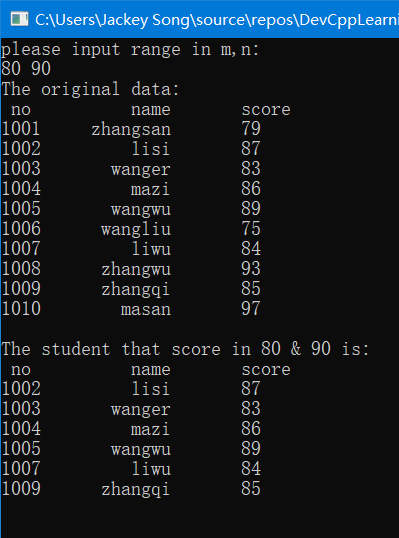结构体类型指针 和 指向结构体数组的指针
指向结构体变量的指针的定义
struct 结构体类型名 *指针名;
struct student stu;
struct student *ps=&stu;

利用结构体指针引用结构体变量成员
指针变量->结构体成员名;
取內容符*，*指针变量表示指针指向的变量，
也可以(*指针变量).成员名
所以有三种方法可以指向结构体成员变量：
结构体变量名 . 成员变量名
指向结构体变量的指针变量的名字 -> 成员变量名
(*指向结构体变量的指针变量的名字) . 成员变量名，括号不可省略
"."是分量运算符

指向结构体数组的指针
struct student s;
struct student *ps=s;//结构体变量名“s”为结构体数组的首地址

也可以写成：
struct student *ps=&s;

查找符合条件的人数(二)
结构体数组作为函数的实参时，可以用指针变量作为形参来接收结构体数组的内存地址，进而进行操作，把上面的代码改用指针来做，如下：(用指针做会更麻烦一些，但是为了加深对指针的印象和应用，我还是用指针做了一遍)
#include <stdio.h>
#define N 10
struct student
{
int no;
char name;
int score;
};
int fun(struct student *p,struct student *p2,int m,int n);//用指针来接收结构体数组的内存地址
void output(struct student *p,int n);//用指针来接收结构体数组的内存地址
int main()
{
struct student s[N]=
{
{1001,"zhangsan",79},
{1002,"lisi",87},
{1003,"wanger",83},
{1004,"mazi",86},
{1005,"wangwu",89},
{1006,"wangliu",75},
{1007,"liwu",84},
{1008,"zhangwu",93},
{1009,"zhangqi",85},
{1010,"masan",97},
};
int m,n,k;
struct student a[N];
printf("please input range in m,n:\n");
scanf("%d%d",&m,&n);
printf("The original data:\n");
output(s,N);
k=fun(s,a,m,n);
printf("The student that score in %d & %d is:\n",m,n);
output(a,k);
return 0;
}
int fun(struct student *p1,struct student *p2,int m,int n)//用指针来接收结构体数组的内存地址
{
int i,k=0;
for(i=0;i<N;i++)
{
if((p1+i)->score>=m&&(p1+i)->score<=n)//用指针指向结构体数组中的人成员变量
*(p2+k++)=*(p1+i);//结构体变量之间的赋值
//注意k++和++k之间的区别
}
return k;
}
void output(struct student *p,int n)//用指针来接收结构体数组的内存地址
{
int i;
printf(" no          name       score\n");
for(i=0;i<n;i++)
printf("%d   %10s       %d\n",(p+i)->no,(p+i)->name,(p+i)->score);
putchar('\n');
}typedef类型
typedef的使用形式:typedef 标准类型名 别名;
如：
typedef int INTEGER;

为标准类型int定义一个别名，可以使用INTEGER来定义变量
INTEGER a,b;

结构体也可以使用typedef类型定义：
typedef struct student
{
int no;
char name;
int score;
}STU;

这样就可以使用STU来定义结构体变量：
STU s1,s2;
STU s;

学生成绩排序
输入n(n<=50)个学生的成绩信息，按照学生的个人平均成绩从高到低输出他们的信息。
#include <stdio.h>
struct student
{
int num;
char name;
int computer,english,math;
double average;
};
int main()
{
int i,index,j,n;
struct student stu,temp;

printf("Input n:");
scanf("%d",&n);
for(i=0;i<n;i++)
{
printf("Input the info of No.%d:\n",i+1);
printf("number:");
scanf("%d",&stu[i].num);
printf("name:");
scanf("%s",stu[i].name);
printf("math score:");
scanf("%d",&stu[i].math);
printf("english score:");
scanf("%d",&stu[i].english);
printf("computer score:");
scanf("%d",&stu[i].computer);
stu[i].average=(stu[i].math+stu[i].english+stu[i].computer)/3.0;
}
for(i=0;i<n-1;i++)
{
index=i;
for(j=i+1;j<n;j++)
if(stu[j].average>stu[index].average)
index=j;
temp=stu[index];
stu[index]=stu[i];
stu[i]=temp;
}
printf("num\tname\taverage\n");
for(i=0;i<n;i++)
printf("%d\t%s\t%.2lf\n",stu[i].num,stu[i].name,stu[i].average);

return 0;
}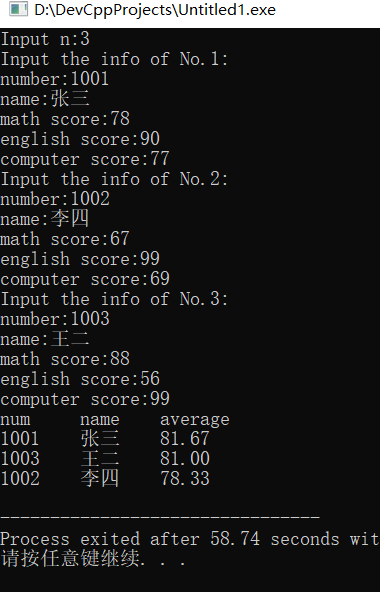修改学生成绩
输入n(n<=50)个学生的成绩信息，再输入一个学生的学号、课程以及成绩，在自定义函数中修改该学生指定课程的成绩
#include <stdio.h>
struct student
{
int num;
char name;
int computer,english,math;
double average;
};
int updata_score(struct student *p,int n,int num,int course,int score);
int main()
{
int course, i, n, num, pos, score;
struct student stu;
printf("Input n:");
scanf("%d",&n);
for(i=0;i<n;i++)
{
printf("Input the info of No.%d:\n",i+1);
printf("number:");
scanf("%d",&stu[i].num);
printf("name:");
scanf("%s",stu[i].name);
printf("math score:");
scanf("%d",&stu[i].math);
printf("english score:");
scanf("%d",&stu[i].english);
printf("computer score:");
scanf("%d",&stu[i].computer);
}
printf("Input the number of the students to be updata:");
scanf("%d",&num);
printf("Choise the course: 1.math  2.english  3.computer:");
scanf("%d",&course);
printf("Input the new score:");
scanf("%d",&score);
pos=updata_score(stu,n,num,course,score);
if(pos==-1)
printf("Not found!\n");
else
{
printf("After updata:\n");
printf("num\tmath\tenglish\tcomputer\n");
printf("%d\t%d\t%d\t%d\n",stu[pos].num, stu[pos].math, stu[pos].english, stu[pos].computer);
}
return 0;
}
int updata_score(struct student *p,int n,int num,int course,int score)
{
int i,pos;
for(i=0;i<n;i++,p++)
if(p->num==num)
break;
if(i<n)
{
switch(course)
{
case 1:p->math=score;break;
case 2:p->english=score;break;
case 3:p->computer=score;break;
}
pos=i;
}
else
pos=-1;
return pos;
}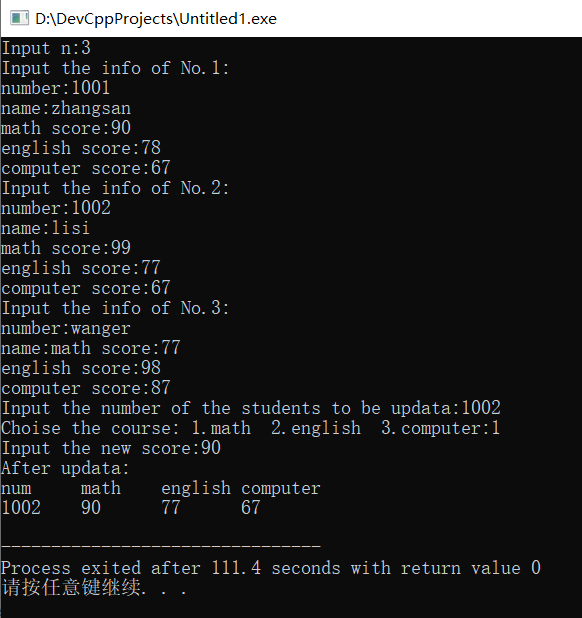下一篇文章
动态数组的实现，结合指针与结构体实现链表，以及链表相关操作


展开全文• 指向结构体变量的指针与指向结构体数组的指针在使用时的区别就是：指向结构体变量的指针，在使用时这样写：struct rsol v;struct rsol *m;m = &amp;v;指向结构体数组的指针，在使用时这样写：struct edc hj={...
指向结构体变量的指针与指向结构体数组的指针在使用时的区别就是：指向结构体变量的指针，在使用时这样写：struct rsol v;struct rsol *m;m = &v;指向结构体数组的指针，在使用时这样写：struct edc hj={1,2,3,4,5};struct edc *k;k = hj;因为结构体数组hj本身就是一个地址（数组的起始地址），所以在使用时不加‘&’，而结构体变量m本身在使用时同普通变量一样，因此要取得m的地址，就得用&m。
展开全文• 例 用指向结构体的指针变量输出结构体数组的元素。 #include&amp;lt;stdio.h&amp;gt; struct student {long num; char name; char sex; float score; }; struct student s={ {97015,&quot;...

指针可以处理数组或数组元素，指向结构体类型的指针也可以处理结构体数组及元素

例  用指向结构体的指针变量输出结构体数组的元素。
#include<stdio.h>
struct student
{long num;
char name;
char sex;
float score;
};
struct student s={
{97015,"WangFang",'F',95.5},
{97018,"LiMing",'M',89.5},
{97045,"ZhangLong",'M',85},
{97026,"ZhaoYue",'F',76}
};
int main()
{int i;
struct student *p;
p=s;
printf("Number Name        Sex   Score\n");
for (i=0;i<4;i++,p++)
{printf("%d  %-13s %-c  %.1f\n",p->num,p->name,p->sex,p->score);
}
return 0;
}

运行结果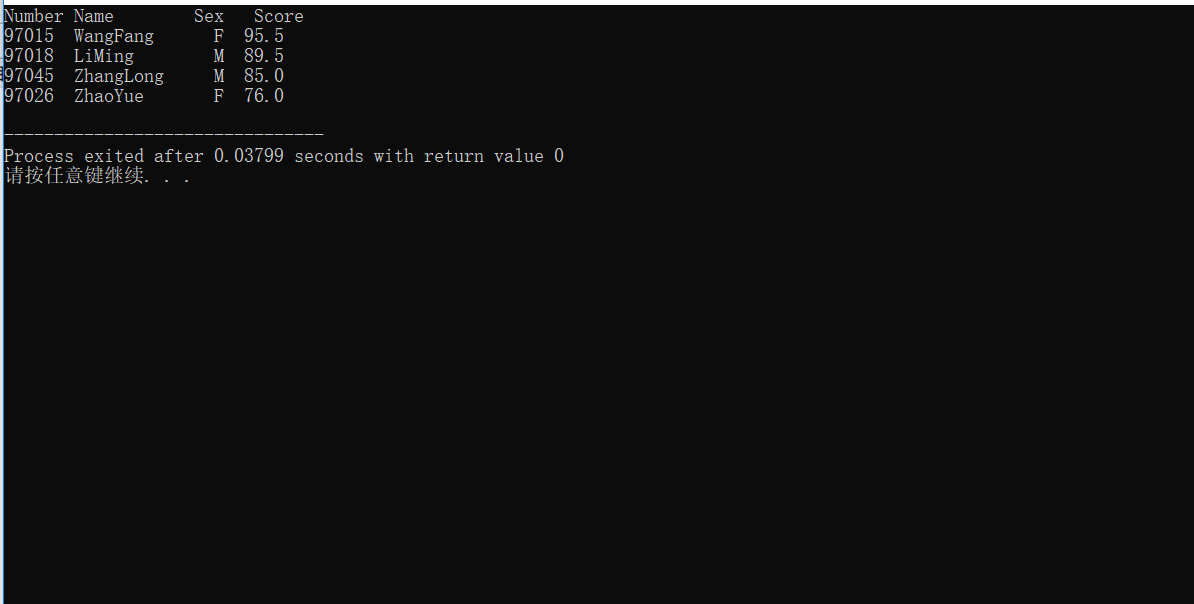程序中的p是指向struct student类型数据的指针变量，将数组s的首地址赋给p，p就是指向s.
下一篇  指针18（ 指针与结构体类型2–指向结构体数组的指针下）


展开全文C语言
• 当结构体指针变量指向一个结构体变量数组的时候，此时指针变量的值就是结构体数组的首地址 关于如何定义结构体数组，和将结构体指针指向结构体变量数组，不是重点。 重点是,明白结构体指针的是怎么移动的， 我个人...
• [中国寒龙联盟出品]C语言基础知识第十三课“指向结构体的指针与链表”指向结构体变量的指针变量，指向结构体数组的指针变量，结构体数据作函数的参数，链表的概念，链表的操作,更多欢迎朋友们的支持！~请关注我们的...
• 题目描述 定义一个结构体student，存储学生的学号、名字、性别和年龄，读入每个学生的所有信息，保存在结构体中，并输出。...本题要求使用指向结构体数组的指针进行输入和输出。 输入 第一行有一个整数n，表示...
• 设ps为指向结构数组的指针变量，则ps也指向该结构数组的0号元素，ps+1指向1号元素，ps+i则指向i号元素。这与普通数组的情况是一致的。 【例11-6】用指针变量输出结构数组。 struct stu{
• 设ps为指向结构数组的指针变量，则ps也指向该结构数组的0号元素，ps+1指向1号元素，ps+i则指向i号元素。这与普通数组的情况是一致的。 【例11-6】用指针变量输出结构数组。 struct stu{ inc语言 struct
• 用3个学生信息，放在结构体类型数组中，要求： 输出全部学生信息 # include<stdio.h> struct Student // 声明结构体类型 struct Student { long num; char name; char sex; int age; }; struct...c语言 编程语言
• 设ps为指向结构数组的指针变量，则ps也指向该结构数组的0号元素，ps+1指向1号元素，ps+i则指向i号元素。这与普通数组的情况是一致的。【例11-6】用指针变量输出结构数组。 1 struct stu 2 { 3......